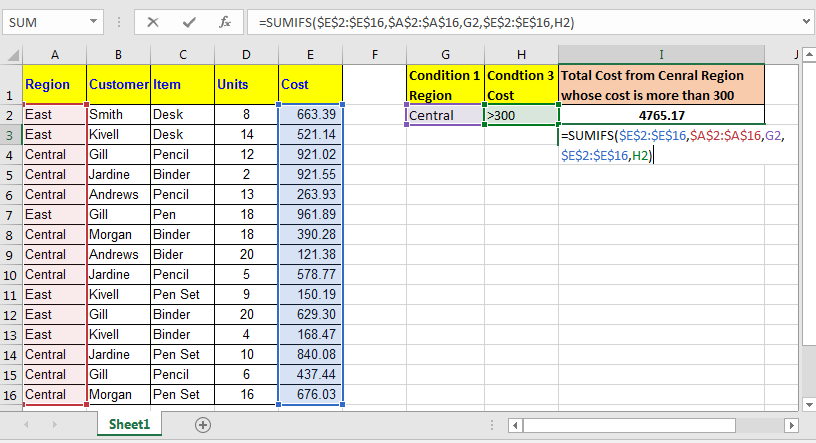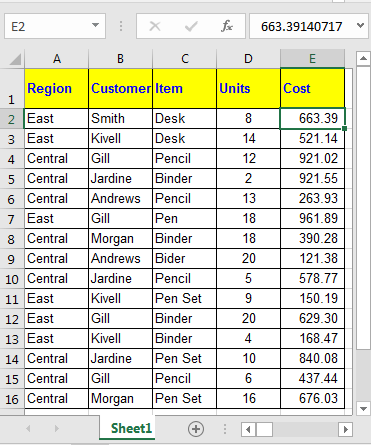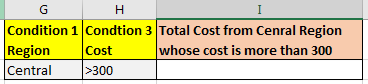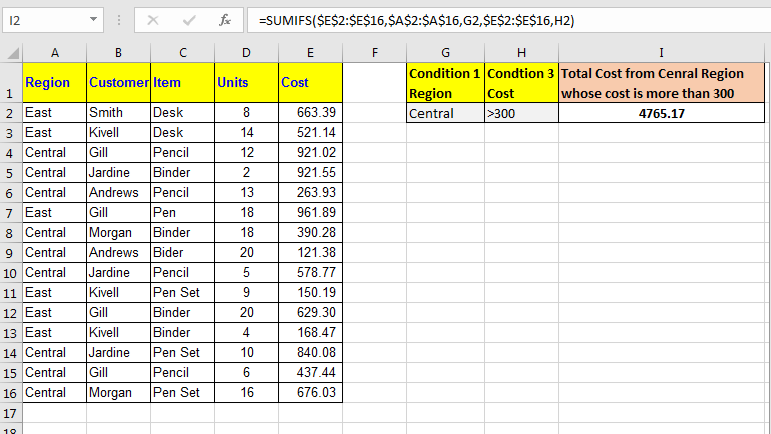# How to Use SUMIFS Function in Excel

The difference between SUMIF and SUMIFS is that SUMIF function sums a range on single condition and the SUMIFS function can sum values on multiple conditions.SUMIFS syntax

=SUMIFS(sum_range, criteria_range1, criteria1, [criteria_range2, criteria2],..... )

Sum_range : The array that you want to sum (must be numeric)

Criteria_range1 : The range of your first condition.

Criteria1 : The condition from Criteria_range1

[Criteria_range2] : The range of your second condition.

[Criteria2] : The condition from Criteria_range2

SUMIFS function works with range-criteria pair. First range-condition pair is mandatory.
Other range-condition pairs are optional but if you give a range than condition is mandatory. Let’s see an example.

#### SUMIFS Example

Lets take the example of a data from stationary store. See the image below.Now we need to get some of Cost of Central region, where Cost is more than 300.Write this SUMIFS formula in excel 2016, 2013, 2010 or older. Its available in all versions of Excel running now.

=SUMIFS(\$E\$2:\$E\$16,\$A\$2:\$A\$16,G2,\$E\$2:\$E\$16,H2)#### Explanation”

\$E\$2:\$E\$16 : This is our sum range. I have used absolute referencing here using \$ sign. It locks your range and does not change when copied to other cell.

\$A\$2:\$A\$16 : The first criteria range 1. Used absolute referencing here too.

G2: its the reference to criteria. G2 contains central, it means “central” is criteria.

\$E\$2:\$E\$16: Its the criteria range 2.

H2: It is the reference to criteria which is “>300”

Its done. You can see the sum of Cost from Cenral Region whose cost is more than 300 in cell I2. Its 4765.17.

If you want sum of pencil units sold to gill than write this SUMIFS formula

=SUMIFS(D2:D16,B2:B16,"Gill",C2:C16,"pencil")
• Note that SUMIFS is not case sensitive.

Sum of units sold to in central to Andrews that are 20 units

=SUMIFS(D2:D16,A2:A16,"Central",B2:B16,"Gill",D2:D16,20)
• Nothing stops you from putting a condition from sum_range itself.

#### PRO NOTES:

• SUMIFS function in excel 2016 can handle 127 range-criteria pairs.
• SUMIFS supports wild card operators (*,?)
• You need to use “” for criteria until and unless you are looking for a numeric match

Related Articles:

SUMIFS using AND-OR logic

SUMIFS with dates in Excel

3 SUMIF with Or Formulas

How to Sum Multiple Columns with Condition

Popular Articles:

50 Excel Shortcuts to Increase Your Productivity

How to use the VLOOKUP Function in Excel

How to use the COUNTIF function in Excel 2016

How to Use SUMIF Function in Excel

Terms and Conditions of use

The applications/code on this site are distributed as is and without warranties or liability. In no event shall the owner of the copyrights, or the authors of the applications/code be liable for any loss of profit, any problems or any damage resulting from the use or evaluation of the applications/code.